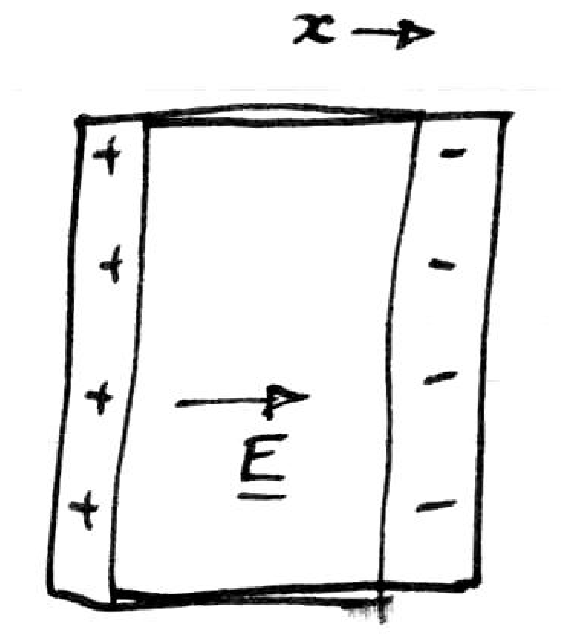Tag Archives: Higgs Boson

Plasmons, the Coulomb Interaction and a Gap

In a famous 1962 paper entitled Plasmons, Gauge Invariance and Mass (pdf!), P.W. Anderson described the relationship between the gap in the plasmon spectrum and the idea of spontaneous symmetry breaking. It is an interesting historical note that Higgs cites Anderson’s paper in his landmark paper concerning the Higgs mechanism.

While there are many different formulations, including Anderson’s, of why the plasmon is gapped at zero momentum in a 3D solid, they all rely on one crucial element: the long-range nature of the Coulomb interaction (i.e. the electrons are charged particles). Of these formulations, I prefer one “cartoon-y” explanation which captures the essential physics well.

Before continuing, let me stress that it is quite unusual for a fluid medium (such as the electrons in a metal) to possess no zero frequency excitations at long wavelengths. For instance,  the dispersion relation for surface gravity waves on water (pdf!) is:$\omega^2(k)=gk \tanh kh$.

Now, in 3D and in the long-wavelength limit, the plasmon sets up opposite charges on the surfaces of the solid as pictured below:The long-wavelength plasmon therefore sets up the same electric field as in a capacitor. The electric field for a capacitor is$\textbf{E} = \frac{\sigma\hat{x}}{\epsilon_0}$. This expression is surprisingly independent of the distance separating the surfaces of the solid. Therefore, it takes a finite amount of energy to set up this electric field, even in the limit of infinite distance. This finite energy results in the gapping of the plasmon.

This cartoon can be extended further to 2D and 1D solids. In the 2D case, the electric field for the 1D “lines of charge” bounding the solid falls off like$\textbf{E}\sim\frac{1}{\textbf{r}}$. Therefore, in the infinite distance limit, it takes no energy to create this electric field and the plasmon is not gapped at$\textbf{q}=0$. Similarly, for the 1D case, the electric field from the points bounding the solid falls of as$\frac{1}{\textbf{r}^2}$, and the plasmon is again gapless.

This reasoning can be applied further to the phenomenon known as LO-TO splitting in a polar solid. Here, the longitudinal optical phonon (LO) and the transverse optical phonon (TO) branches are non-degenerate down to the very lowest (but non-zero!) momenta. Group theory predicts these modes to be degenerate at$\textbf{q}=0$ for the zincblende crystal structure of typical semiconducting compounds. Below is the phonon dispersion for GaAs demonstrating this phenomenon:Again, the splitting occurs due to the long-ranged nature of the Coulomb interaction. In this case, however, it is the polar ionic degree of freedom that sets up the electric field as opposed to the electronic degrees of freedom. Using the same reasoning as above, one would predict that the LO-TO splitting would disappear in the 2D limit, and a quick check in the literature suggests this to be the case as reported in this paper about mono-layer Boron Nitride.

I very much appreciate toy models such as this that give one enough physical intuition to be able to predict the outcome of an experiment. It has its (very obvious!) limitations, but is valuable nonetheless.

Was the Higgs Boson Discovered in 1980?

In a tour-de-force experiment in 1980, Klein and Sooryakumar discovered a collective mode in the superconducting phase of NbSe$_2$ in a Raman experiment. This mode interacts with the collective mode of the charge density wave and was interpreted a few year later by Littlewood and Varma to be a Higgs amplitude mode of the superconducting state.

Could it really be said that the Higgs mode was discovered in Urbana in 1980?

Of course, I am being facetious, but it is a rather cute historical curiosity. That being said, I have to also admit that I am not 100% convinced that this interpretation is correct, though it does carry some weight.

This classic experiment has also been driving experiments recently towards observing the Higgs mode in other superconducting systems as well. For example, click here and here.

The other part of this experiment that makes it particularly relevant to current studies is the observation of an interaction between superconductivity and charge density waves as was mentioned in a previous post. With the application of a magnetic field, they were able to suppress the superconductivity and enhance the charge density wave collective excitation as pictured below.